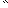MINLPLib

A Library of Mixed-Integer and Continuous Nonlinear Programming Instances

Instance: ex5_4_2

 Formatsⓘ ams gms lp mod nl osil pip Primal Bounds (infeas ≤ 1e-08)ⓘ 7512.23014500 p1 ( gdx sol ) (infeas: 0) Other points (infeas > 1e-08)ⓘ Dual Boundsⓘ 7512.23011500 (ANTIGONE)7512.23013700 (BARON)7512.23014200 (COUENNE)7512.23014400 (LINDO)7512.23014400 (SCIP) Referencesⓘ Floudas, C A, Pardalos, Panos M, Adjiman, C S, Esposito, W R, Gumus, Zeynep H, Harding, S T, Klepeis, John L, Meyer, Clifford A, and Schweiger, C A, Handbook of Test Problems in Local and Global Optimization, Kluwer Academic Publishers, 1999.Avriel, M and Williams, A C, An Extension of Geometric Programming with Applications in Engineering Optimization, Journal of Engineering Mathematics, 5:2, 1971, 187-194. Sourceⓘ Test Problem ex5.4.2 of Chapter 5 of Floudas e.a. handbook Added to libraryⓘ 31 Jul 2001 Problem typeⓘ QCP #Variablesⓘ 8 #Binary Variablesⓘ 0 #Integer Variablesⓘ 0 #Nonlinear Variablesⓘ 8 #Nonlinear Binary Variablesⓘ 0 #Nonlinear Integer Variablesⓘ 0 Objective Senseⓘ min Objective typeⓘ linear Objective curvatureⓘ linear #Nonzeros in Objectiveⓘ 3 #Nonlinear Nonzeros in Objectiveⓘ 0 #Constraintsⓘ 6 #Linear Constraintsⓘ 3 #Quadratic Constraintsⓘ 3 #Polynomial Constraintsⓘ 0 #Signomial Constraintsⓘ 0 #General Nonlinear Constraintsⓘ 0 Operands in Gen. Nonlin. Functionsⓘ Constraints curvatureⓘ indefinite #Nonzeros in Jacobianⓘ 17 #Nonlinear Nonzeros in Jacobianⓘ 8 #Nonzeros in (Upper-Left) Hessian of Lagrangianⓘ 10 #Nonzeros in Diagonal of Hessian of Lagrangianⓘ 0 #Blocks in Hessian of Lagrangianⓘ 3 Minimal blocksize in Hessian of Lagrangianⓘ 2 Maximal blocksize in Hessian of Lagrangianⓘ 3 Average blocksize in Hessian of Lagrangianⓘ 2.666667 #Semicontinuitiesⓘ 0 #Nonlinear Semicontinuitiesⓘ 0 #SOS type 1ⓘ 0 #SOS type 2ⓘ 0 Infeasibility of initial pointⓘ 1.225e+06 Sparsity JacobianⓘSparsity Hessian of Lagrangianⓘ\$offlisting
*
*  Equation counts
*      Total        E        G        L        N        X        C        B
*          7        1        0        6        0        0        0        0
*
*  Variable counts
*                   x        b        i      s1s      s2s       sc       si
*      Total     cont   binary  integer     sos1     sos2    scont     sint
*          9        9        0        0        0        0        0        0
*  FX      0
*
*  Nonzero counts
*      Total    const       NL      DLL
*         21       13        8        0
*
*  Solve m using NLP minimizing objvar;

Variables  x1,x2,x3,x4,x5,x6,x7,x8,objvar;

Equations  e1,e2,e3,e4,e5,e6,e7;

e1..  - x1 - x2 - x3 + objvar =E= 0;

e2..    x4 + x6 =L= 400;

e3..  - x4 + x5 + x7 =L= 300;

e4..  - x5 + x8 =L= 100;

e5.. x1 - x1*x6 + 833.333333333333*x4 =L= 83333.3333333333;

e6.. x2*x4 - x2*x7 - 1250*x4 + 1250*x5 =L= 0;

e7.. x3*x5 - x3*x8 - 2500*x5 =L= -1250000;

* set non-default bounds
x1.lo = 100; x1.up = 10000;
x2.lo = 1000; x2.up = 10000;
x3.lo = 1000; x3.up = 10000;
x4.lo = 10; x4.up = 1000;
x5.lo = 10; x5.up = 1000;
x6.lo = 10; x6.up = 1000;
x7.lo = 10; x7.up = 1000;
x8.lo = 10; x8.up = 1000;

Model m / all /;

m.limrow=0; m.limcol=0;
m.tolproj=0.0;

\$if NOT '%gams.u1%' == '' \$include '%gams.u1%'

\$if not set NLP \$set NLP NLP
Solve m using %NLP% minimizing objvar;

Last updated: 2021-11-30 Git hash: 8337812f